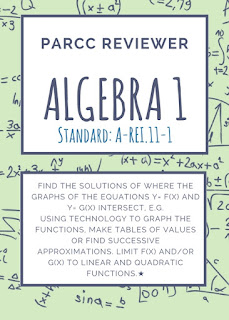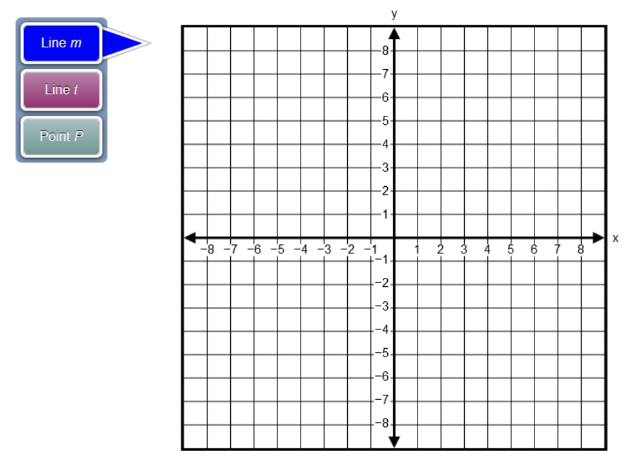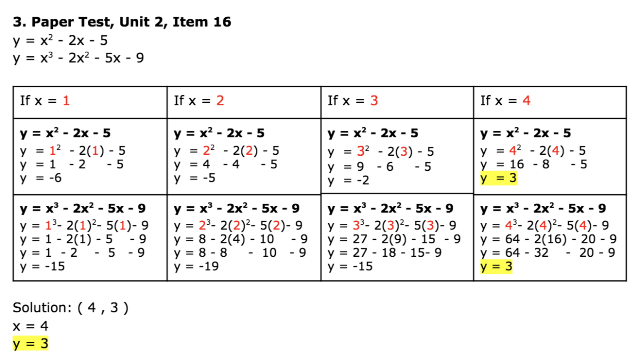## Labels

### Algebra 1 PARCC Reviewer 10 (A-REI.11-1)

Sub-claim: A
The students solve problems involving the Major Content with connections to the Standards for Mathematical Practice
Type of items: Type 1
Color Code: Peach
Evidence Statement Key: A-REI.11-1
Calculator: Y
Y = Yes; Assessed on Calculator Section
X = Calculator is Specific to Item
N = No; Assessed on Non-Calculator Sections
Z = Calculator Neutral (Could be Calculator or Non-Calculator Sections)
Point: 1 each (1:26)
Evidence Statement Text:

Find the solutions of where the graphs of the equations y= f(x) and y= g(x) intersect, e.g. using technology to graph the functions, make tables of values or find successive approximations. Limit f(x) and/or g(x) to linear and quadratic functions.★
Clarifications, limits, emphases, and other information intended to ensure appropriate variety in tasks:

i) The "explain" part of standard A-REI.11 is not assessed here. For this aspect of the standard, see Sub-Claim C.
Sample Questions (taken from PARCC’s Practice Tests and Released Items):

PBA Released Test Item 4
EOY Released Test Item 29
Practice Test, Unit 2, Item 2
Paper Test, Unit 2, Item 161. PBA Released Test Item 4
Graph lines m and t on the xy-coordinate plane shown. Then plot the point of intersection P.
Line m : y = -2/3 x - 4
Line t : y = x + 6
To graph a line, select two points on the coordinate plane. A line will be drawn through the points. Select the place on the coordinate plane to plot the point.2. EOY Released Test Item 29 The graph of f(x) = -x3 - 4 and g(x) = 0.5x2 + x + 4 are given.Use the graphs to find the solution to the equation
-x3 -4 = 0.5x2 + x + 4.
Answers: